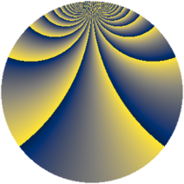# Properties

 Label 3630.2.bpLevel $3630$ Weight $2$ Character orbit 3630.bp Rep. character $\chi_{3630}(29,\cdot)$ Character field $\Q(\zeta_{110})$ Dimension $10560$ Sturm bound $1584$

# Related objects

## Defining parameters

 Level: $$N$$ $$=$$ $$3630 = 2 \cdot 3 \cdot 5 \cdot 11^{2}$$ Weight: $$k$$ $$=$$ $$2$$ Character orbit: $$[\chi]$$ $$=$$ 3630.bp (of order $$110$$ and degree $$40$$) Character conductor: $$\operatorname{cond}(\chi)$$ $$=$$ $$1815$$ Character field: $$\Q(\zeta_{110})$$ Sturm bound: $$1584$$

## Dimensions

The following table gives the dimensions of various subspaces of $$M_{2}(3630, [\chi])$$.

Total New Old
Modular forms 32000 10560 21440
Cusp forms 31360 10560 20800
Eisenstein series 640 0 640

## Trace form

 $$10560q - 264q^{4} - 12q^{9} + O(q^{10})$$ $$10560q - 264q^{4} - 12q^{9} - 22q^{10} + 264q^{16} + 20q^{25} + 30q^{30} - 20q^{31} - 8q^{34} - 8q^{36} - 20q^{39} + 10q^{40} - 48q^{45} + 20q^{46} + 296q^{49} + 108q^{51} + 68q^{55} + 40q^{61} - 264q^{64} - 44q^{66} + 24q^{69} + 14q^{70} + 48q^{75} - 68q^{79} - 124q^{81} + 20q^{84} + 60q^{85} + 90q^{90} - 320q^{91} + 80q^{94} + 124q^{99} + O(q^{100})$$

## Decomposition of $$S_{2}^{\mathrm{new}}(3630, [\chi])$$ into newform subspaces

The newforms in this space have not yet been added to the LMFDB.

## Decomposition of $$S_{2}^{\mathrm{old}}(3630, [\chi])$$ into lower level spaces

$$S_{2}^{\mathrm{old}}(3630, [\chi]) \cong$$ $$S_{2}^{\mathrm{new}}(1815, [\chi])$$$$^{\oplus 2}$$